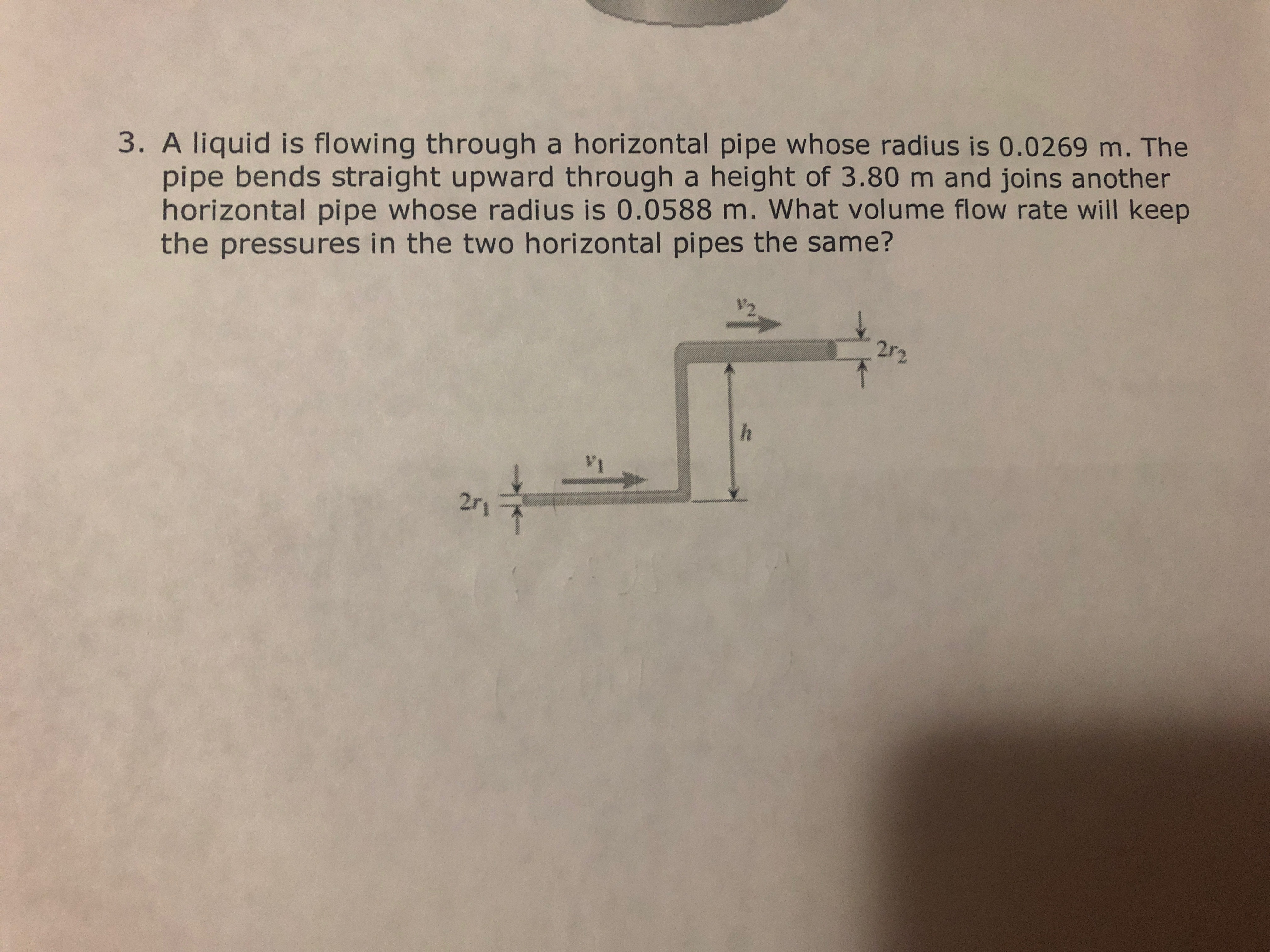# 3. A liquid is flowing through a horizontal pipe whose radius is 0.0269 m. Thepipe bends straight upward through a height of 3.80 m and joins anotherhorizontal pipe whose radius is 0.0588 m. What volume flow rate will keepthe pressures in the two horizontal pipes the same?2r-2

Question
87 viewshelp_outlineImage Transcriptionclose3. A liquid is flowing through a horizontal pipe whose radius is 0.0269 m. The pipe bends straight upward through a height of 3.80 m and joins another horizontal pipe whose radius is 0.0588 m. What volume flow rate will keep the pressures in the two horizontal pipes the same? 2r-2 fullscreen
check_circle

Step 1

Given that the radius of the bottom pipe is r1=0.0269m, the radius of the top pipe is r2=0.0588m, and the height h=3.80m. The cross sectional areas of the bottom pipe and the top pipe can be computed as shown below.

Step 2

Let v­1 and v2 be the speed of flow in the bottom pipe and top pipe respectively. The equation of continuity of the flow can be written as shown below.

Step 3

Write the Bernoulli’s equa...

### Want to see the full answer?

See Solution

#### Want to see this answer and more?

Solutions are written by subject experts who are available 24/7. Questions are typically answered within 1 hour.*

See Solution
*Response times may vary by subject and question.
Tagged in

### Physics